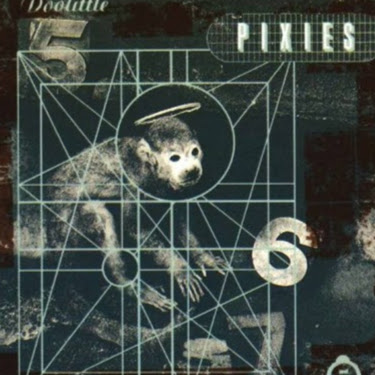5:29 AM
@PrateekMourya if you're around ping me.

1 hour later…6:47 AM
@JohnRennie Hey@psa hi :-)just got a quick question, i think it just follows from the classical multiplication rule but wanted to check
just part (d)
I said that $|\Psi(x_1,x_2,t)|^2 = |\Psi_1(x_1,t)\Psi_2(x_2,t)|^2$ and I think that the pdf of the product of two independent random variables is just the product of the pdfs, so $|\Psi_1(x_1,t)\Psi_2(x_2,t)|^2 = |\Psi_1(x_1,t)|^2|\Psi_2(x_2,t)|^2$ hence $|\Psi(x_1,x_2,t)|^2 = |\Psi_1(x_1,t)|^2|\Psi_2(x_2,t)|^2$I'm not sure to be honest. My memory of probability is too vague for me to be sure whether that's correct or not.I'm not sure if it's totally accurate to say that the wavefunction is a classical random variable, though, but yeah.
no worries(d) seems an odd question.
The previous parts of the question nicely explains why the SE factors into a part for each particle.6:54 AM
in any case, that implies $|\Psi| = |\Psi_1||\Psi_2|$ (taking the positive square roots without any loss of generality). however I'm not sure that it follows then that $\Psi = \Psi_1\Psi_2$?
I tried converting the modulus to be in terms of the complex conjugate but I don't think that helps. maybe it trivially follows, idk.I would ask in the h bar. There are plenty of hard core QMers there.ok :)
thanks !
ah actually no
converting to complex conjugate shows it's trivial
from $|\Psi|^2$
yeah that was an interesting question. it's cool that it adds so nicely like that. i was reading feynman's exposition on the hydrogen atom and then he started trying to show the equations for Helium and Z above.
it got too complicated mathematically but he was able to show how the patterns show up in the periodic table anyways, qualitatively.7:14 AM
Here one of them is an octahedral void and one tetrahedral
How do I identify which is which
@JohnRennie@user586228 you have to look at the 3D structure.Can you share a schematic to support this
@JohnRennie I actually cannot relate the two..That is where the problem lies
I am exceptionally bad at 3D to 2D transition.
So I seek helpokHave a look at that and see if it helps.
The nice thing about videos is they can do the 3D animations to show exactly what the voids look like.7:39 AM
Thanks a lot @JohnRennie Sir:-)

3 hours later…10:42 AM
@napstablook KYPY is over?31jan
I got this weird overconfidence in physics somehow and negative marking is sucking all my marks in mocksthen what exam was this from?past papersnapstablook : "i was awarded bonus"that was probably a typo10:53 AM
hmm okor they probably meant getting bonus when marking the past paper after doing it11:37 AM
How do I contruct a right angle triangle to generate a quadratic and find out the radius of the maximum sphere that can fir in the void?

1 hour later…12:54 PM
distance bw centres > 2 radius
you dont need to contruct right triangles always, constructing those are prefered because it becomes euclidean geometry instead of all the rearranging involved in coordinate1:38 PM
I do not think so @RishiNandhaVanchi
Letus wait for @JohnRennie

1 hour later…2:48 PM
sure, why not